# Sequence

In the arithmetic sequence is a1=-1, d=4. Which member is equal to the number 203?

n =  52

### Step-by-step explanation: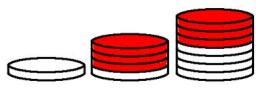Did you find an error or inaccuracy? Feel free to write us. Thank you!Tips to related online calculators
Do you have a linear equation or system of equations and looking for its solution? Or do you have a quadratic equation?

## Related math problems and questions:

• SequenceIn the arithmetic sequence is given: Sn=2304, d=2, an=95 Calculate a1 and n.
• SequenceWrite the first 7 members of an arithmetic sequence: a1=-3, d=6.
• Probably memberLook at the series 2,6,25,96,285, ? What number should come next?
• Geometric sequenceIn the geometric sequence is a4 = 20 a9 = -160. Calculate the first member a1 and quotient q.
• Nineteenth memberFind the nineteenth member of the arithmetic sequence: a1=33 d=5 find a19
• Gauss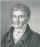Help little C.F. Gauss sum all the integers from 1 to 400.
• Sequence 11What is the nth term of this sequence 1, 1/2, 1/3, 1/4, 1/5?
• Special sequence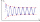What if            2×9=1            3×9=2            4×9=3            5×9=4            6×9=5            7×9=6            8×9=7            9×9=8           10×9=9    then 1×9=??? answer with solutions. .. ..
• Sequence 2Write the first 5 members of an arithmetic sequence a11=-14, d=-1
• Three ints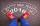The sum of three consecutive integers is 2016. What numbers are they?
• Digits A, B, CFor the various digits A, B, C is true: the square root of the BC is equal to the A and sum B+C is equal to A. Calculate A + 2B + 3C. (BC is a two-digit number, not a product).
• Diofant equationIn the set of integers (Z) solve the equation: 212x +316y =0 Write result with integer parameter t in Z (parameter t = ...-2,-1,0,1,2,3... if equation has infinitely many solutions)
• Diofant equation250x + 120y = 5640
• Progression-12, 60, -300,1500 need next 2 numbers of pattern
• Two-digit numberIn a two-digit number, the number of tens is three greater than the number of units. If we multiply the original number by a number written in the same digits but in reverse order, we get product 3 478. Find the original number.
• Three numbers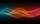The sum of the three numbers is 287. These numbers are in a ratio of 3: 7: 1/4. Find these numbers.
• n-gon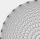Gabo draws n-gon, which angles are consecutive members of an arithmetic sequence. The smallest angle is 70° biggest 170°. How many sides have Gabo's n-gon?